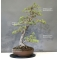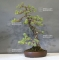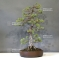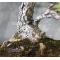##### The Japanese Bonsai specialist
Direct order Contact Help / Services Newsletter# Pinus pentaphylla bonsai ref: 26090131

Pinus pentaphyllaref. : 8055Product unavailable

###### Description

Height of the tree: 68 cm.

Length of the branches: 56 cm.

Non-enamelled brown pot: Ø 32*8 cm.

Ø of the trunk: 6/7 cm.

One of our masterpieces, this pinus pentaphylla or five needle pine is more than 40 years old grown from a seedling. The sokan style tree has beautiful bark, an established root base, a good conical shape trunk and no major wounds or traces of cut branches. It must be kept in full sun.

#bonsai 4.6 #masterpieces 4.2 #pentaphylla 4.1 #pinus 3.5 #branches 2.8 #trunk 2.5 #tree 2.4 #sokan style 2.2 #established 2.1 #beautiful 1.9

Formule
(( ROUND((CHAR_LENGTH(b.article_nom)-CHAR_LENGTH(REPLACE(b.article_nom, 'pentaphylla', '')))/LENGTH('pentaphylla')) + ROUND((CHAR_LENGTH(b.article_description)-CHAR_LENGTH(REPLACE(b.article_description, 'pentaphylla', '')))/LENGTH('pentaphylla')) ) * 4.1) + (( ROUND((CHAR_LENGTH(b.article_nom)-CHAR_LENGTH(REPLACE(b.article_nom, 'pinus', '')))/LENGTH('pinus')) + ROUND((CHAR_LENGTH(b.article_description)-CHAR_LENGTH(REPLACE(b.article_description, 'pinus', '')))/LENGTH('pinus')) ) * 3.5) + (( ROUND((CHAR_LENGTH(b.article_nom)-CHAR_LENGTH(REPLACE(b.article_nom, 'branches', '')))/LENGTH('branches')) + ROUND((CHAR_LENGTH(b.article_description)-CHAR_LENGTH(REPLACE(b.article_description, 'branches', '')))/LENGTH('branches')) ) * 2.8) + (( ROUND((CHAR_LENGTH(b.article_nom)-CHAR_LENGTH(REPLACE(b.article_nom, 'bonsai', '')))/LENGTH('bonsai')) + ROUND((CHAR_LENGTH(b.article_description)-CHAR_LENGTH(REPLACE(b.article_description, 'bonsai', '')))/LENGTH('bonsai')) ) * 2.6) + (( ROUND((CHAR_LENGTH(b.article_nom)-CHAR_LENGTH(REPLACE(b.article_nom, 'trunk', '')))/LENGTH('trunk')) + ROUND((CHAR_LENGTH(b.article_description)-CHAR_LENGTH(REPLACE(b.article_description, 'trunk', '')))/LENGTH('trunk')) ) * 2.5) + (( ROUND((CHAR_LENGTH(b.article_nom)-CHAR_LENGTH(REPLACE(b.article_nom, 'tree', '')))/LENGTH('tree')) + ROUND((CHAR_LENGTH(b.article_description)-CHAR_LENGTH(REPLACE(b.article_description, 'tree', '')))/LENGTH('tree')) ) * 2.4) + (( ROUND((CHAR_LENGTH(b.article_nom)-CHAR_LENGTH(REPLACE(b.article_nom, 'sokan style', '')))/LENGTH('sokan style')) + ROUND((CHAR_LENGTH(b.article_description)-CHAR_LENGTH(REPLACE(b.article_description, 'sokan style', '')))/LENGTH('sokan style')) ) * 2.2) + (( ROUND((CHAR_LENGTH(b.article_nom)-CHAR_LENGTH(REPLACE(b.article_nom, 'masterpieces', '')))/LENGTH('masterpieces')) + ROUND((CHAR_LENGTH(b.article_description)-CHAR_LENGTH(REPLACE(b.article_description, 'masterpieces', '')))/LENGTH('masterpieces')) ) * 2.2) + (( ROUND((CHAR_LENGTH(b.article_nom)-CHAR_LENGTH(REPLACE(b.article_nom, 'established', '')))/LENGTH('established')) + ROUND((CHAR_LENGTH(b.article_description)-CHAR_LENGTH(REPLACE(b.article_description, 'established', '')))/LENGTH('established')) ) * 2.1) + (( ROUND((CHAR_LENGTH(b.article_nom)-CHAR_LENGTH(REPLACE(b.article_nom, 'enamelled', '')))/LENGTH('enamelled')) + ROUND((CHAR_LENGTH(b.article_description)-CHAR_LENGTH(REPLACE(b.article_description, 'enamelled', '')))/LENGTH('enamelled')) ) * 1.9)

## Secure payment## Delivery

Our logistic partners :04 74 55 23 48
Pépinière MAILLOT-BONSAÏ
Le Bois Frazy
01990 RELEVANT - FRANCE
on appointmentThe Japanese Bonsai specialist
If you like Maillot Bonsaï, share on  facebook

A vos côtés. Les services restent les mêmes.

La vente continue et les expéditions également.

Meilleurs vœux pour 2021.

Guy Maillot

OK